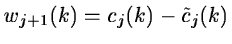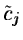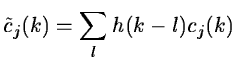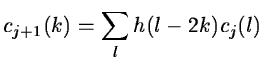Next: Multiresolution with scaling functions Up: Pyramidal Algorithm Previous: The Laplacian Pyramid

### Pyramidal Algorithm with one Wavelet

To modify the previous algorithm in order to have an isotropic wavelet transform, we compute the difference signal by:(14.43)

butis computed without reducing the number of samples:(14.44)

and cj+1 is obtained by:(14.45)

The reconstruction method is the same as with the laplacian pyramid, but the reconstruction is not exact. However, the exact reconstruction can be performed by an iterative algorithm. If P0 represents the wavelet coefficients pyramid, we look for an image such that the wavelet transform of this image gives P0. Van Cittert's iterative algorithm gives:

 Pn+1 = P0 + Pn - R(Pn) (14.46)

where
• P0 is the pyramid to be reconstructed
• Pn is the pyramid after n iterations
• R is an operator which consists in doing a reconstruction followed by a wavelet transform.
The solution is obtained by reconstructing the pyramid Pn.

We need no more than 7 or 8 iterations to converge. Another way to have a pyramidal wavelet transform with an isotropic wavelet is to use a scaling function with a cut-off frequency.Next: Multiresolution with scaling functions Up: Pyramidal Algorithm Previous: The Laplacian Pyramid
Petra Nass
1999-06-15